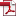• KSII Transactions on Internet and Information Systems
Monthly Online Journal (eISSN: 1976-7277)

# Joint Optimization Algorithm Based on DCA for Three-tier Caching in Heterogeneous Cellular Networks

Vol. 15, No. 7, July 31, 202110.3837/tiis.2021.07.019, Download Paper (Free):#### Abstract

In this paper, we derive the expression of the cache hitting probability with random caching policy and propose the joint optimization algorithm based on difference of convex algorithm (DCA) in the three-tier caching heterogeneous cellular network assisted by macro base stations, helpers and users. Under the constraint of the caching capacity of caching devices, we establish the optimization problem to maximize the cache hitting probability of the network. In order to solve this problem, a convex function is introduced to convert the nonconvex problem to a difference of convex (DC) problem and then we utilize DCA to obtain the optimal caching probability of macro base stations, helpers and users for each content respectively. Simulation results show that when the density of caching devices is relatively low, popular contents should be cached to achieve a good performance. However, when the density of caching devices is relatively high, each content ought to be cached evenly. The algorithm proposed in this paper can achieve the higher cache hitting probability with the same density.